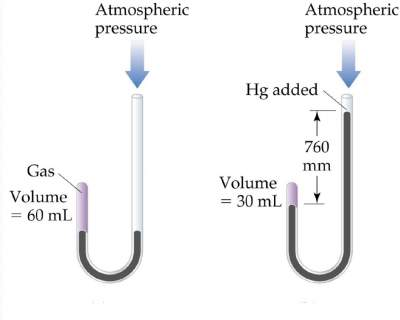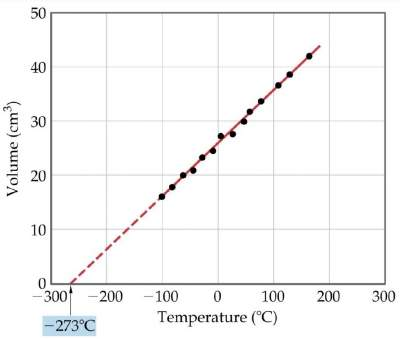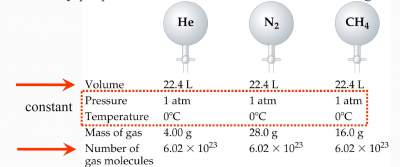# Gas Laws

## Boyle's Law

"Volume of a fixed quantity of gas is inversely proportional to its pressure"

 V = constant × 1/P PV = constant## Charles' Law

"The volume of a fixed quantity of gas at constant pressure increases as the temperature Increases"
 V = constant × T V / T = constantNote:
When temperature is measured in °C, the intercept on the temperature axis is − 273.15°C (called absolute zero)

"The volume of a gas at a given temperature and pressure is directly proportional to the number of moles of the gas"
V = constant × nBased on the three laws, we obtain V = constant × nT/P

Hence the Ideal Gas Law, V = R × nT/P →

## Ideal Gas Law

PV = nRT

Where R is gas constant and its value depends on the units of P, V, T used.

STP (Standard Temperature & Pressure)are: T: 0°C; 273.15 K, P: 1 atm.
Volume of 1 mol of gas at STP is:
V = nRT/P = (1 mol)(0.08206 L·/mol·K)(273.15K)/ 1.000 atm = 22.4 L

For an ideal gas under 2 sets of conditions:
P1V1 = n1RT1 & P2V2 = n2RT2
Hence, R = P1V1 / n1T1 & R = P2V2 / n2T2
P1V1 / n1T1 = P2V2 / n2T2

Rearranging the gas law yields: n/V = P/RT
Multiply by the molar mass (M): nM/V = PM/RT
Since nM = w in grams)
(Gas density) d = PM/RT → (Molar Mass) M = dRT/P

### Gas mixtures and partial pressures

"In a gas mixture the total pressure is given by the sum of partial pressures of each component"

Hence, Ptotal = P1 + P2 + P1 + ....

### Dalton's Law

Remark: If each gas obeys the ideal gas equation:
Pi = n(RT/V) where Pi is called Partial Pressure.
Ptotal = (n1 + n2 + n3 + ....)(RT/V)

If ni is the number of moles of gas (i) exerting a partial pressure
Pi = Xi Ptotal
where Xi (mole fraction) = ni/ntotal

### Exercises on Gas Laws

Exercise I on Finding Total Pressure

Check your answers here: Solution to the Exercise I on Finding Total Pressure

Exercise II on Finding Total Pressure

Check your answers here: Solution to the Exercise II on Finding Total Pressure

Exercise III on Finding Total Pressure

Check your answers here: Solution to the Exercise III on Finding Total Pressure